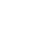# 图论：最小费用流与Bellman-Ford算法

## 最小传输费用问题

Bellman-Ford模板：

```#define N 1000
#define INF 100000000

struct Edge
{
int from,to,cap,flow,cost;
};
struct MCMF
{
int n,m,s,t;
vectoredges;
vectorG
;
int inq
;//是否在队列中
int d
;//Bellman-Ford
int p
;//上一条弧
int a
;//可改进量

void init(int n)
{
this->n=n;
for(int i=0;i<n;i++)
G[i].clear();
edges.clear();
}
void addedge(int from,int to,int cap,int cost)
{
edges.push_back((Edge){from,to,cap,0,cost});
edges.push_back((Edge){to,from,0,0,-cost});
m=edges.size();
G[from].push_back(m-2);
G[to].push_back(m-1);
}

bool BellmanFord(int s,int t,int&flow,long long&cost)//沿着最短路增广
{
for(int i=0;i<n;i++)
d[i]=INF;
memset(inq,0,sizeof(inq));
d[s]=0,inq[s]=1,p[s]=0,a[s]=INF;

queueq;
q.push(s);
while(!q.empty())
{
int u=q.front();q.pop();
inq[u]=0;
for(int i=0;i<G[u].size();i++) { Edge&e=edges[G[u][i]]; if(e.cap>e.flow&&d[e.to]>d[u]+e.cost)//松弛操作
{
d[e.to]=d[u]+e.cost;
p[e.to]=G[u][i];//记录父边
a[e.to]=min(a[u],e.cap-e.flow);//更新可改进量，等于min{到达u时候的可改进量，e边的残量}
if(!inq[e.to]){q.push(e.to);inq[e.to]=1;}
}
}
}
if(d[t]==INF)//仍为初始时候的INF，s-t不连通，失败退出
return false;
flow+=a[t];
cost+=(long long)d[t]*a[t];//d[t]一方面代表了最短路长度，另一方面代表了这条最短路的单位费用的大小
int u=t;
while(u!=s)//逆向修改每条边的流量值
{
edges[p[u]].flow+=a[t];
edges[p[u]^1].flow-=a[t];
u=edges[p[u]].from;
}
return true;
}

int MincostMaxflow(int s,int t,long long&cost)//返回最大流，同时用引用返回达到最大流时的最小费用
{
int flow=0;
cost=0;
while(BellmanFord(s,t,flow,cost));//直到不存在最短路时停止
return flow;
}
};
```

## 特殊情况

• 对于流量任意
如果需要计算包含负权边的图中流量任意但费用最小的流。可以根据最小流算法中h[i+1](v)>=h[i](v)的性质，在h[i](t)<0时进行增广。
• 对于费用为负
存在负权边需要用Bellman-Ford算法。存在负圈可以利用算法找到负圈并在负圈上尽量增广。通过变形避免负权边的算法（每次增广时如边数相等，通过对所有边费用加上合适常数将边转成非负数）只适用部分情况。

## 《图论：最小费用流与Bellman-Ford算法》有2个想法

1.linanwy

同学，你的代码被转义了，请看第二十五行

回复
1.落絮飞雁 文章作者

谢谢，已经改了。

回复